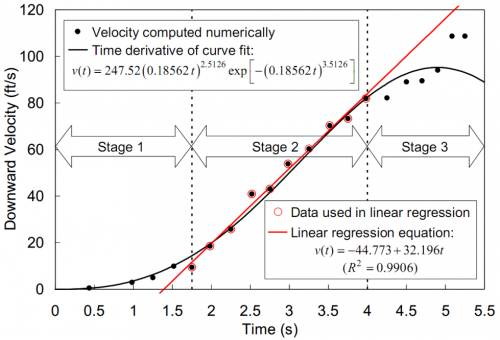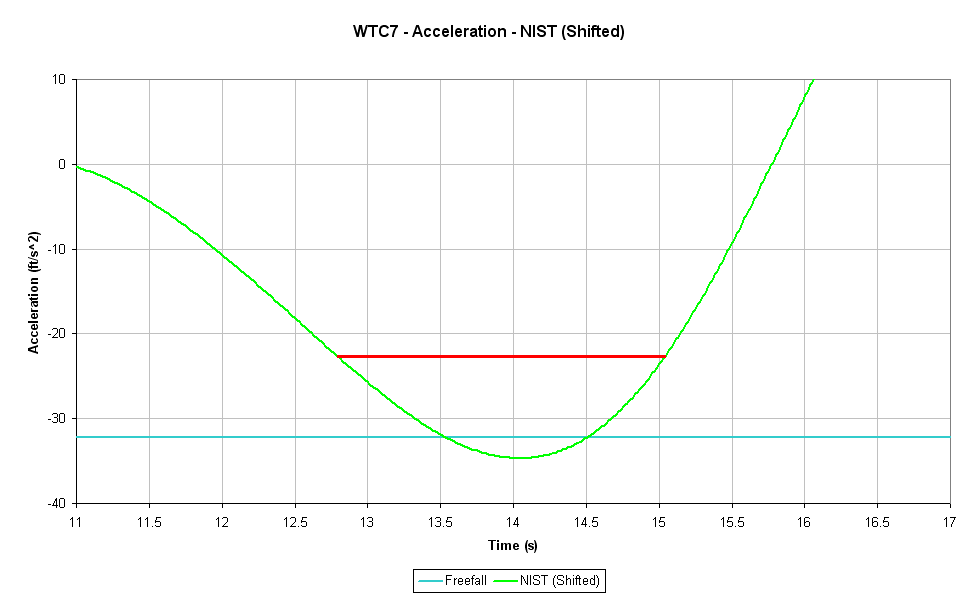Thread: [Merged] Discussion of femr's video data analysis View Single Post6th May 2011, 06:47 AM #1214 femr2 Illuminator   Join Date: Apr 2009 Posts: 3,859 Originally Posted by W.D.Clinger The red line is NIST's linear approximation to part of NIST's nonlinear approximation. ... You were telling us that your [acceleration] polynomials must be better than NIST's [acceleration] approximations because (you thought) NIST's [acceleration] approximations were linear. ... you didn't realize that NIST's [acceleration] approximations were nonlinear ... NIST's model of the acceleration is not a straight line. ... NIST's models of displacement, velocity, and acceleration are all nonlinear. ... NIST's velocity and acceleration models are immediate consequences (via freshman calculus) of that nonlinear model. ... etc Parentheses added by me for clarity of context. A slight reminder on this point... NIST only derived their displacement formula to velocity. The only acceleration model used is the linear regression, and it is from that linear regression that their stated metrics are generated...NIST used the datapoints circled in red (which were themselves determined by central difference approximation from the underlying displacement data) to calculate their stated linear regression (the red line)... v(t) = -44.773 + 32.196t It is this linear regression which is used to specify the rough 2.25s *freefall* period. That fact is made even clearer if we actually look at the implied NIST acceleration function (which they did not use) and check the period of time which it can reasonably be said to approximate gravitational acceleration...I have taken the liberty of marking a 2.25s duration in red and gravitational acceleration in cyan. __________________ http://the911forum.freeforums.org Last edited by femr2; 6th May 2011 at 08:01 AM.